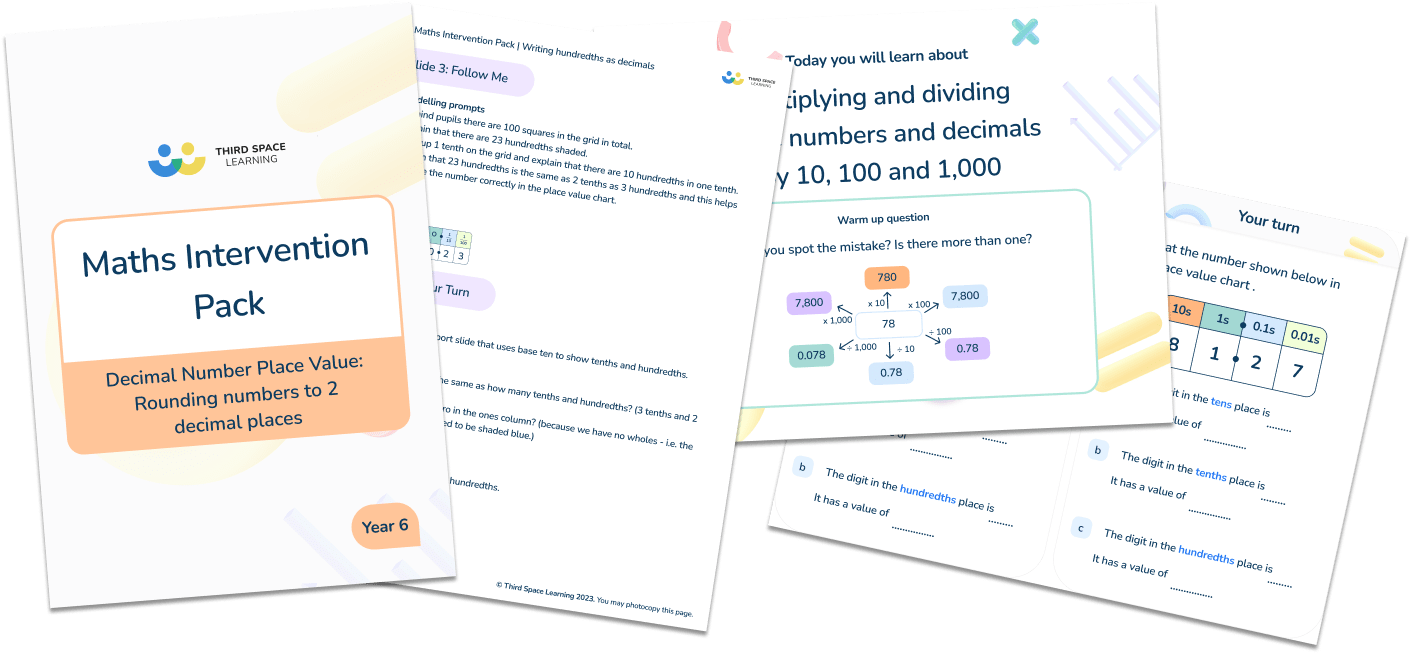# Maths Intervention Pack Decimal Place Value

### Years 3, 4, 5 and 65 intervention lessons on decimal place value to help your pupils develop their knowledge and understanding of decimal place value.

• Lessons include:
• Writing hundredths as decimals (Year 4)
• Ordering numbers with up to 2 decimal places (Year 5)
• Value digits in numbers with up to 2 decimal places (Year 5)
• Multiplying and dividing by 10,100 and 1,000 (Year 6)
• Rounding numbers to 2 decimal places (Year 6)
• Each lesson contains teaching notes, answers and slides to work through with your pupils.
• Lessons follow exactly the same format that the Third Space Learning specialist maths tutors use in their one to one online maths tutoring.